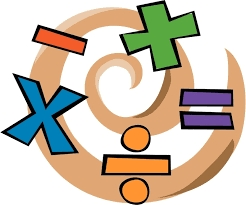# Differing 7391

The product of two numbers differing by four does not change when we decrease the larger number by two and increase the smaller number by one. What are the numbers?

a =  6
b =  2

### Step-by-step explanation:Did you find an error or inaccuracy? Feel free to write us. Thank you!

Tips for related online calculators
Are you looking for help with calculating roots of a quadratic equation?
Do you have a linear equation or system of equations and looking for its solution? Or do you have a quadratic equation?